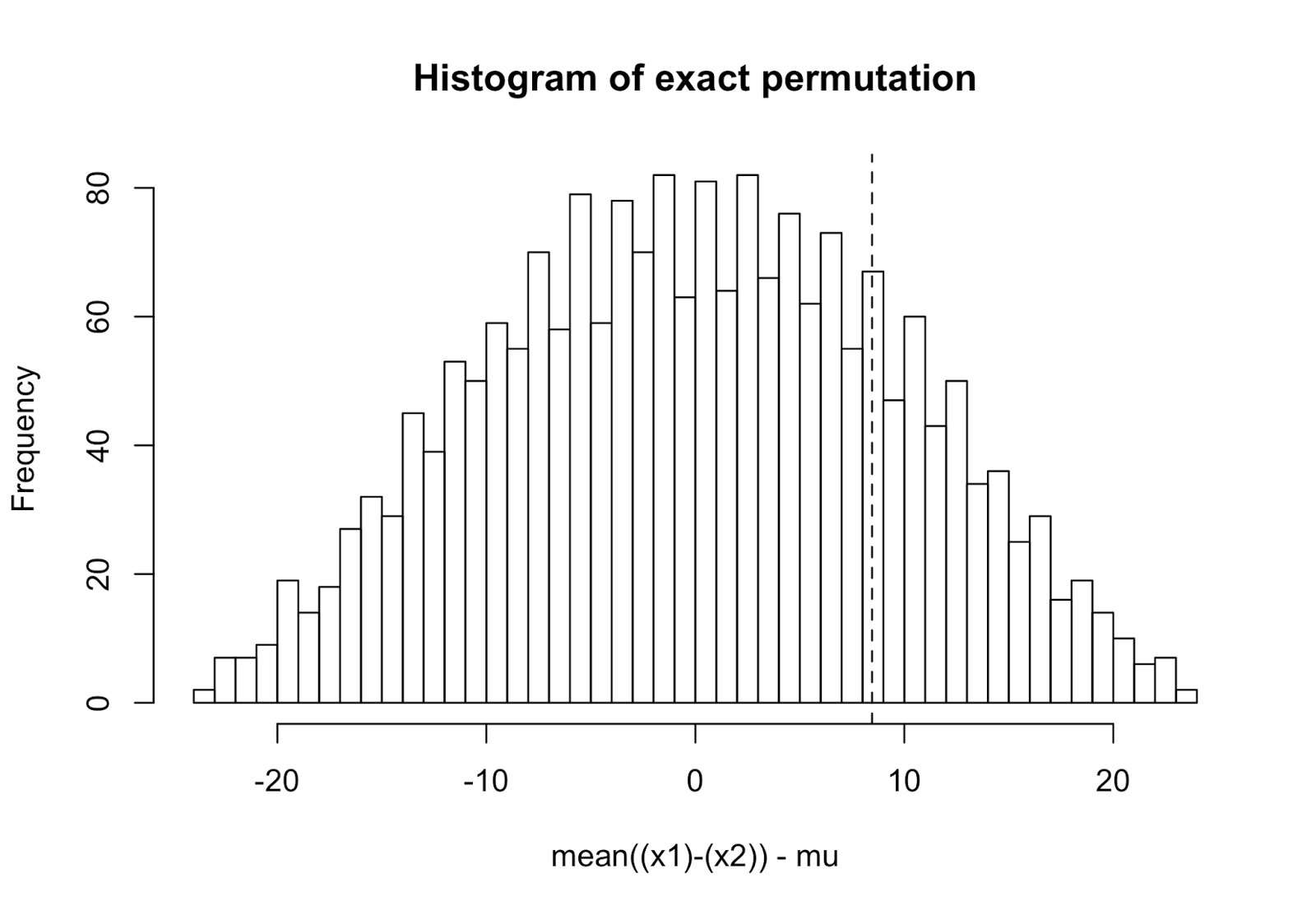## Pages

### 統計檢驗簡介：一個二項式分配的簡單例子

※ 引述《mrlee112233 (小史)》之銘言：
: 不好意思
: 我想問一下顯著水準
: 有沒有簡單一點的解釋
: 我上網查過資料
: 但因爲我不是相關科系
: 所以我跟本看不懂在寫什麼
: 虛無假設.type l error .type ll error..等
: 跟本不懂=   =
: 我文獻報告裏
: 他假設alpha=0.05 然後用二相分佈去做計算
: 所以想問有沒有比較淺顯易懂的解釋
: 謝謝


### An R function: Exact one/paired sample test for mean(s)

Here I provide a exact one sample or paired sample test for mean(s), which is the exact version of permutation test to compare a single sample against a mean or to compare paired samples’ differences against a mean.

The algorithm in this function is based on Bryan F. J. Manly. 1997. Randomization, bootstrap and Monte Carlo methods in biology. 2nd edition. pp. 91-97. That is, all possible permutations (i.e. all possible cases of exchange between $x_i$ and $\mu$ in one-sample test or all possible cases of exchange between $x_{1i}$ and $x_{2i}$) in paired-sample test are processed to calculate a exact p-value.

#### Arguments

The usage of this function exactOneOrPairedSampleTest() is very similar to the R built-in function t.test(). There are four arguments:

• x1: a numeric vector specifying $x_{1i}$
• x2 = NULL: a numeric vector specifying $x_{2i}$ (in paired-sample case)
• mu = 0: a number specifying true mean or true difference $\mu$
• alternative = c("t","g","l")a single character specifying $H_0$: true mean of $x_1$ or mean of $x_{1i} - x_{2i}$ is equal, greater or less than $\mu$, respectively.

#### Example 1: one-sample test

Let $x_i = \{43,67,64,64,51,53,53,26,36,48,34,48,6 \}$, $i=1 \ldots 13$:

> x <- c(43,67,64,64,51,53,53,26,36,48,34,48,6)

Now we can call the above function exactOneOrPairedSampleTest() to test $H_0$: true mean of $x_i = 56$ against $H_A$: true mean of $x_i \neq 56$:

> test1 <- exactOneOrPairedSampleTest(x, alternative="t", mu=56)
> test1

Exact one sample test

Alternative hypothesis: true mean is not equal to 56

mean(x) - mu = -10.3846153846154
Number of total permutation = 8192
Number of rejected permutation = 364
P-value = 0.04443359

The results show that:

• $\sum_{i=1}^{13} x_i / 13 - \mu = 45.61538 - 56 = -10.38462$;
• Totally $8192 = 2^{13}$ permutations are processed;
• 364 permutations do not support $H_0$;
• $P = 364/2^{13} = 0.0444$.

We can also call the function hist.exactOneOrPairedSampleTest():

> hist(test1)As you can see, the vertical lines $x=\pm 10.38462$ shows the critical boundary of rejecting $H_0$. Note that it is a two-tail test.

Finally, you may call the returned object:

> str(test1)
List of 14
$x1 : num [1:13] 43 67 64 64 51 53 53 26 36 48 ...$ x2          : NULL
$x1.name : chr "x"$ x2.name     : chr "NULL"
$n : int 13$ mu          : num 56
$test.0 : num -10.4$ is.onesample: logi TRUE
$alternative : chr "t"$ test.perm   : num [1:8192] -10.38 -8.38 -12.08 -10.08 -11.62 ...
$DF :'data.frame': 13 obs. of 3 variables: ..$ x1  : num [1:13] 43 67 64 64 51 53 53 26 36 48 ...
..$mu : num [1:13] 56 56 56 56 56 56 56 56 56 56 ... ..$ diff: num [1:13] -13 11 8 8 -5 -3 -3 -30 -20 -8 ...
$N : num 8192$ p.value     : num 0.0444
$rejected.N : int 364 - attr(*, "class")= chr "exactOneOrPairedSampleTest" to get more details of the results if you need them. #### Example 2: paired-sample test Let$x_{1i} = \{92, 0,72,80,57,76,81,67,50,77,90\}$and$x_{2i} = \{43,67,64,64,51,53,53,26,36,48,34\}$, where each$i$is paired and$i=1 \ldots 11$: > x1 <- c(92, 0,72,80,57,76,81,67,50,77,90) > x2 <- c(43,67,64,64,51,53,53,26,36,48,34) Now we can call the function exactOneOrPairedSampleTest() to test$H_0$: true mean of$x_{1i} - x_{2i} \leq 10$against$H_A$: true mean of$x_{1i} - x_{2i} > 10$: > test2 <- exactOneOrPairedSampleTest(x1, x2, alternative="g", mu=10) > # equivalent to test2 <- exactOneOrPairedSampleTest(x1 - x2, alternative="g", mu=10) > test2 Exact paired sample test Alternative hypothesis: means of x1 - x2 is greater than 10 mean((x1)-(x2)) - mu = 8.45454545454546 Number of total permutation = 2048 Number of rejected permutation = 445 P-value = 0.2172852  The results show that: •$\sum_{i=1}^{11} (x_{1i}-x_{2i}) / 11 - 10 = 8.45455$; • Totally$2048 = 2^{11}$permutations are processed; • 445 permutations do not support$H_0$; •$P = 445/2^{11} = 0.2172852$. We can also call the function hist.exactOneOrPairedSampleTest(): > hist(test2)As you can see, the vertical lines$x=8.45455$shows the critical boundary of rejecting$H_0$. Note that this is a right-tail test. Again, you may call the returned object: > str(test2) List of 14$ x1          : num [1:11] 92 0 72 80 57 76 81 67 50 77 ...
$x2 : num [1:11] 43 67 64 64 51 53 53 26 36 48 ...$ x1.name     : chr "x1"
$x2.name : chr "x2"$ n           : int 11
$mu : num 10$ test.0      : num 8.45
$is.onesample: logi FALSE$ alternative : chr "g"
$test.perm : num [1:2048] 8.45 1.36 22.45 15.36 8.82 ...$ DF          :'data.frame': 11 obs. of  3 variables:
..$x1 : num [1:11] 92 0 72 80 57 76 81 67 50 77 ... ..$ x2  : num [1:11] 43 67 64 64 51 53 53 26 36 48 ...
..$diff: num [1:11] 39 -77 -2 6 -4 13 18 31 4 19 ...$ N           : num 2048
$p.value : num 0.217$ rejected.N  : int 445
- attr(*, "class")= chr "exactOneOrPairedSampleTest"

to get more details of the results if you need them.